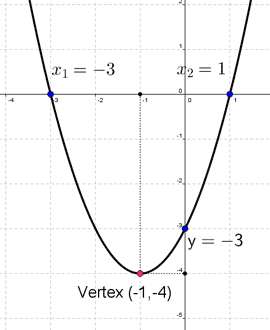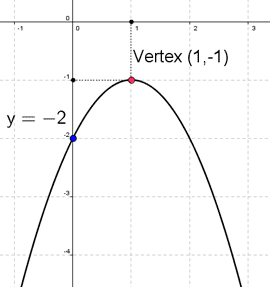Math Calculators, Lessons and Formulas

It is time to solve your math problem

mathportal.org

This calculator draws the quadratic function and finds the x and y intercepts, vertex, and focus. For each of these calculations, the calculator will generate a full explanation.

Graphing functions of the form $y = ax^2 + bx + c = 0$
show help ↓↓ examples ↓↓
Hide steps
working...
examples
example 1:ex 1:
Graph quadratic function: $y = \frac{x^2}{2} - 3x - 4$.
example 2:ex 2:
Graph the function $f(x) = x^2 + 0.5x - \frac{2}{3}$.
example 3:ex 3:
Graph the function $f(x) = - x^2 + \frac{5}{4}$.

Quadratic function has the form $f(x) = ax^2 + bx + c$ where a, b and c are numbers

You can sketch quadratic function in 4 steps. I will explain these steps in following examples.

Example 1:

Sketch the graph of the quadratic function

$${\color{blue}{ f(x) = x^2+2x-3 }}$$

Solution:

In this case we have $a=1, b=2$ and $c=-3$

STEP 1: Find the vertex.

To find x - coordinate of the vertex we use formula:

$$x=-\frac{b}{2a}$$

So, we substitute $1$ in for $a$ and $2$ in for $b$ to get

$$x=-\frac{b}{2a} = -\frac{2}{2\cdot1} = -1$$

To find y - coordinate plug in $x=-1$ into the original equation:

$$y = f(-1) = (-1)^2 + 2\cdot(-1) - 3 = 1 - 2 - 3 = -4$$

So, the vertex of the parabola is ${\color{red}{ (-1,-4) }}$

STEP 2: Find the y-intercept.

To find y - intercept plug in $x=0$ into the original equation:$$f(0) = (0)^2 + 2\cdot(0) - 3 = 0 - 0 - 3 = -3$$

So, the y-intercept of the parabola is ${\color{blue}{ y = -3 }}$

STEP 3: Find the x-intercept.

To find x - intercept solve quadratic equation $f(x)=0$ in our case we have:

$$x^2+2x-3 = 0$$

Solutions for this equation are:

$${\color{blue}{ x_1 = -3 }} ~~~\text{and}~~~ {\color{blue}{ x_2 = 1 }}$$

( to learn how to solve quadratic equation use quadratic equation solver )

STEP 4: plot the parabola.

Example 2:

Sketch the graph of the quadratic function

$${\color{blue}{ f(x) = -x^2+2x-2 }}$$

Solution:

Here we have $a=-1, b=2$ and $c=-2$

The x-coordinate of the vertex is:

$${\color{blue}{ x = -\frac{b}{2a} }} = -\frac{2}{2\cdot(-1)}= 1$$The y-coordinate of the vertex is:

$$y = f(1) = -1^2+2\cdot1-2 = -1 + 2 - 2 = -1$$

The y-intercept is:

$$y = f(0) = -0^2+2\cdot0-2 = -0 + 0 - 2 = -2$$

In this case x-intercept doesn't exist since equation $-x^2+2x-2=0$ does not has the solutions (use quadratic equation solver to check ). So, in this case we will plot the graph using only two points

Search our database of more than 200 calculators